## Inductance

Can changing current in a coil induce a current in another coil not connected to it ? Can the changing current in a coil affect its own current ?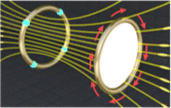## SelfInductance

Changing current in a coil induces an opposing EMF in itself. What does this induced EMF depend on ?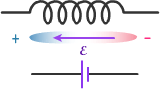## Solenoid

How to find the Inductance of an Ideal Solenoid ?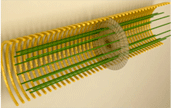## Q1

What will happen to the inductance of a solenoid
a) when it is stretched to twice its length maintaining the same number of turns and cross - sectional area ?
b) when the number of turns and the length are doubled keeping the area of cross section same ?
c) when the air inside the solenoid is replaced by iron of relative permeability mr ?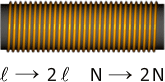## Q2

The length of a wire required to manufacture a solenoid of length l and self - induction L is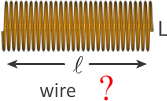## Kirchhoff''sLaw

Is Kirchoff’s Loop Rule valid for Induced EMF ? Because Kirchoff’s Loop Rule is based on Potential difference which is defined only for Conservative fields like Electrostatic Field. And since Induced EMF is due to Induced Electric Field which is not a Conservative Field. So can we treat Induced EMF as Potential Difference ?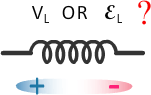"

## Q3

Potential difference across an inductor (L) as function of time is shown in figure. Draw the graph of current through inductor vs time." "

## Q4

a) Find the current through the inductor and p.d across it. Also plot the graphs of current through the inductor and p.d across it wrt time
b) Find the p.d between the points MN, MO, and OP"

## SeriesParallelCombination

Equivalent Inductance of Inductors connected in Series and Parallel Combination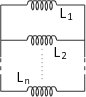## Q5

The equivalent inductance of two inductors is 2.4 H when connected in parallel and 10 H when connected in series. What is the value of inductance of the individual inductors ?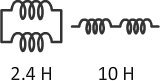## MutualInductance

How does a changing current in one loop induce an EMF in another non-connected loop ? Does a changing current in the second loop also induce an EMF in the first loop ? Are these effects symmetric ?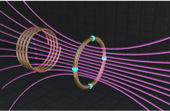"

## Q6

Two coils with resistance R1 and R2 are placed as shown in figure. When there is current in coil 1, then one quarter of the magnetic flux produced by coil 1 links with coil 2 , giving a mutual inductance M. What will be the mutual inductance when coil 2 is used as the primary? How much current should change in Dt in coil 1, so that the induced current in coil 2 is i2 ?" "

## Q7

Two coils are placed at fixed locations near each other. When coil 1 has no current and the current in coil 2 increases at the rate of 10 A/s, then EMF in the coil 1 is 100 mV. When coil 2 has no current and coil 1 has a current of 5 A, then what will be the flux linkage in coil 2 ?" "

## Q8

Two coils shown in figure, have self inductances LA and LB and mutual inductance between them is M. What will be the equivalent inductance of the combination of coils, if
a) A' is connected to B b) A' is connected to B' The coils are now wound on a long plastic tube in the same sense.
What will be the equivalent inductance of the combination of coils, if
a) A' is connected to B
b) A' is connected to B'"

## Q9

Find the inductance (per unit length) of a system of two infinite parallel wires, each of radius 'a', whose centres are a distance 'd' apart and carry equal currents in opposite directions. Neglect the flux within the wire.
Calculate the inductance (per unit length) of a double tape line as shown in figure, if the tapes are separated by a distance 'd' which is considerably less than their length L and width W.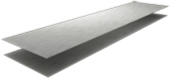## RLCircuit-1

Equation of flow of increasing current in a Series RL circuit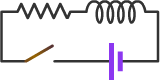## RLCircuit-2

Equation of flow of decreasing current in a Series RL circuit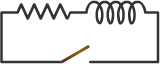## Q10

In the following circuit, the switch is closed at t = 0. Find the equation of current through the inductor and resistor R1 as a function of time.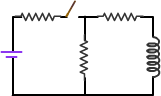## Q11

A circuit consists of an ideal cell, an inductor L and a resistor R, connected in series. Let switch S be closed at t = 0. Suppose at t = 0 the current in the inductor is io, then find out the equation of current as a function of time.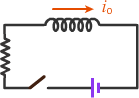## EnergyinRLCircuit

Energy variations in a series RL circuit. Can an inductor store energy like a Capacitor ? What is this energy in the form of ?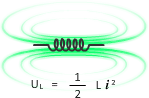"

## Q12

Two different coils have self - inductance L1 and L2 . The current in one coil is increased at a constant rate. The current in the second coil is also increased at the same constant rate. At a certain instant of time, the power given to the two coils is the same. At that time, the current, the induced voltage and the energy stored in the first coil are i1, V1 and U1 respectively. Corresponding values for the second coil at the same instant are i2, V2 and U2 respectively. Then what will be the ratio" "

## Q13

For the circuit shown in figure, the switch S is initially in position 1 for a long time. Find the potential difference VA – VB and the rate of heat dissipation in R1. If now switch S is put in position 2 at t = 0 Find: the equation of current through battery the time when current through battery is half the steady value. iii) the energy stored in the inductor L at that time." "

## Q14

Consider the RL circuit in figure. Let PL be the rate at which energy is stored in the inductor and PR be the rate of energy dissipation in the resistor. Switch is closed at t = 0.
a) Express the ratio PL /PR in terms of the current in circuit and time constant of circuit
b) Express the ratio PL /PR as a function of time
c) Find the time at which PL = PR" "

## Q15

A long coaxial cable consists of two thin-walled concentric conducting cylinders with radii a and b. The inner cylinder carries a steady uniform current i, and the outer cylinder provides the return path for that current. The current sets up a magnetic field between the two cylinders.
a) What is the mutual inductance of the system ?
b) What is the mutual inductance per unit length of the system ?
c) What is the energy stored in the magnetic field for a length l of the cable."

## LCCircuit-1

Equation of flow of current in an LC Circuit.## LCCircuit-2

Energy variations in an LC Circuit. Can electrical systems show oscillations like me mechanical systems like spring-block system ?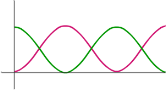## Q16

What will be the natural frequency of oscillation of current in the circuits shown in the figure.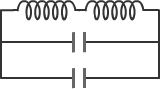"

## Q17

An inductor of inductance 2 mH is connected across a charged capacitor of capacitance 5 mF and the resulting L-C circuit is set oscillating at its natural frequency. Let q denote the instantaneous charge on the capacitor and i the instantaneous current in the circuit. It is found that the maximum value of q is 200 mC.
a) What is the angular frequency and time period of oscillation of circuit ?
b) When q = 100 mC, What is the value of |di / dt| ?
c) What is the maximum value of i ?
d) When i is equal to half its maximum value, what is the value of | q | ?
e) Starting from the time when capacitor is fully charged, after how much time is the energy in capacitor and inductor equal ?" "

## Q18

In an LC circuit shown in figure, C = 1 F , L = 4 H. At time t = 0, charge in the capacitor is 4 C and it is decreasing at the rate of 5 C/s. What will be the maximum charge on capacitor ? What is the phase constant at t = 0 ?"

## Q19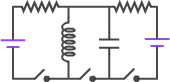"

## Q20

The circuit shown in figure is in steady state with switch S1 closed. At t = 0, S1 is opened and switch S2 is closed.
a) Derive an expression for the charge on capacitor C2 as a function of time.
b) Determine the first instant, when the energy in the inductor becomes half of that in the capacitor." "

## Q21

Initially the capacitor C1 is charged to a potential of V and the capacitor C2 is uncharged. Then switch S1 is closed for time t1 , after which it is opened and at the same instant switch S2 is closed for time t2 and then opened. It is now found that the capacitor C2 is charged to 2V. Find the minimum possible values of the time interval t1 and t2"

## 1

A toroid is wound over a circular core. Radius of each turn is 'r' and radius of toroid is R ( >> r ). Self - inductance of the toroid will be"

## 2

Find the mutual inductance of two concentric coils of radii a1 and a2 ( a1 << a2 )
a) if the coils lie in the same plane b) if the plane of coils make an angle q with each other
c) if the planes of the coils are perpendicular. If the coils are in the same plane and a current i = 2t flows in the smaller loop, then (neglecting self - inductance of the larger loop),
d) find the e.m.f. induced in the larger coil;
e) if the resistance of the larger loop is R, find the current in it as a function of time.
If a capacitor of capacitance C is also connected in the larger loop, find
f) the charge on the capacitor as a function of time.
g) current in the larger loop as a function of time"

## 3

A small loop of wire of side l is placed inside a large square loop of wire of side L ( L >> l ). The loops are coplanar and their centres coincide. The mutual inductance of the system is proportional to"

## 4

Two circular coils can be arranged in any of the three situations shown in the figure. Their mutual inductance will be maximum in which case ?
For case (A), radius of bigger coil is R , and radius of smaller coil is r (r << R). Centre of smaller coil lies on the axis of bigger coil at a distance z from the center of bigger coil and its plane is perpendicular to the axis. Find the mutual inductance between the coils. Find the mutual inductance, if z >> R." "

## 5

In the circuit shown A and B are two cells of same emf E but different internal resistances r1 and r2 ( r1 > r2 ) respectively. Find the value of R such that the potential across the terminals of cell A is zero a long time after the switch S is closed." "

## 6

A coil of wire having inductance and resistance has a co-planar conducting ring placed co-axially within it. The coil is connected to a battery at time
t = 0, so that a time dependent current i1 (t) starts flowing through the coil. If i2 (t) is the current induced in the ring and B(t) is the magnetic field at the center of the coil due to i1 (t) , then the product i2 (t) B(t)
a) increases with time
b) decreases with time
c) does not vary with time
d) passes through a maximum" "

## 7

1. Initially, the capacitor was uncharged. Now, switch S1 is closed and S2 is kept open. If time constant of this circuit is t, then mark the correct statements
a) after time interval t, charge on the capacitor is CV/2
b) after time interval 2t, charge on the capacitor is CV( 1 - e-2)c) the work done by the voltage source will be half of the heat dissipated when the capacitor is fully charged2. After the capacitor gets fully charged, S1 is opened and S2 is closed. Then mark the correct statements
a) at t = 0, energy stored in the circuit is purely in the form of magnetic energy
b) at t > 0, there is no exchange of energy between the inductor and capacitor
c) at any time t > 0, maximum instantaneous current in the circuit may be V C/L"

## 8

In the circuit shown in figure, the battery has negligible internal resistance. Show that the current in the circuit through the battery rises instantly to its steady state value E / R when the switch is closed, provided that resistance R is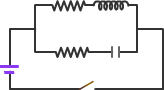## 9

In the given circuit, find the equation of current through the inductor and battery.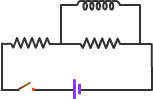## 10

In the circuit shown in figure, S2 remains closed for a long time and S1 is open. Now S1 is also closed. Just after S1 is closed, find the current through each resistor and the rate of change of current through inductor.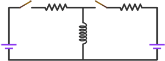## 11

In the circuit shown in figure, initial current through the inductor at t = 0 is io . After a time t = L / R , the switch is quickly shifted to position 2.
a) Plot a graph showing the variation of current with time.
b) Calculate the value of current in the inductor at t =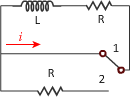"

## 12

In the given circuit, the switch is initially closed for a long time. The switch is thrown open at t = 0.
a) Find the initial voltage Vo across L just after t = 0
b) What is the total energy dissipated in R1 after t = 0 ?" "

## 13

Switch S shown in figure is closed at t = 0. Find the equation of current through each inductor." "

## 14

An inductor of inductance L and resistors of resistance R1 and R2 are connected to a battery of emf E as shown in the figure. The internal resistance of the battery is negligible. The switch S is closed at time t = 0. What is the potential drop across L as a function of time? After the steady state is reached, the switch is opened. What is the direction and the magnitude of current through R1 as a function of time." "

## 15

In the circuit shown, switches S1 and S3 are closed for 1 s while S2 remained open. Just after 1 s, switch S2 is closed and S1 and S3 are opened. After this instant ( switch S2 is closed and S1 and S3 are opened ) , find
a) the maximum current in the circuit
b) the maximum charge on the capacitor
Reconsider the above problem if the polarity of battery B is reversed." "

## 16

An inductor L and a capacitor C are connected in series. Current in the circuit is driven by a device and is given by i = 20 t , where t is in second and i is in amperes. Initially, the capacitor has no charge. Determine
a) the voltage across inductor and capacitor as a function of time
b) the time when the energy stored in the capacitor exceeds that in the inductor"

## 17

In the given circuit, initially switch S1 is closed for a long time, and S2 and S3 are open. At t = 0, S1 is opened and S2 and S3 are closed. If the relation between inductance, capacitance and resistance is L = 4CR2, then find the time after which current passing through capacitor and inductor will be same.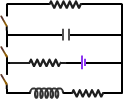"

## 18

An inductor and two capacitors are connected in the circuit as shown in figure. Initially capacitor A has no charge and capacitor B has CV charge. At t = 0, switch S is closed. Find the expression of charge on each capacitor as a function of time.When current in the circuit is maximum,
a) charge on each capacitor is same ?
b) charge on capacitor A is twice the charge on capacitor B ?
c) potential difference across each capacitor is same ?
d) potential difference across capacitor A is twice the charge on capacitor B ?" "

## 19

Two capacitors of capacitance 2C and C, respectively, are connected in series with an inductor of inductance L. Initially the capacitors have charge such that VB - VA = 4Vo and VC - VD = Vo. Initial current in the circuit is zero. Find
a) maximum current that will flow in the current
b) potential difference across each capacitor when current is maximum
c) equation of current in the circuit
d) equation of charge on each capacitor" "

## 20

In the given circuit, S1 remains closed for a long time. Then S2 is closed and S1 is opened. Find out the di/dt in the right loop just after that moment. Also plot the variation of current in the loop and potential difference across the inductor wrt time." "

## 21

Figure shows a part of some circuit. The capacity of the capacitor is 6 mF and is decreasing at the constant rate 0.5 mF/s. Potential difference across the capacitor at the shown moment is changing as follows: The current in the 4 W resistor is decreasing at the rate of 1 mA/s. What is the potential difference across the inductor at this moment?"

## 22

Derive an expression for the total magnetic energy stored in two coils with inductances L1 and L2 and mutual inductance M , when the currents in the coils are I1 and I2 respectively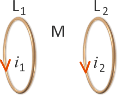"

## 23

A conducting rod of mass 'm' and length l slides over two conducting rails connected to a resistor and inductor as shown in figure. Find the expression of external force required to move it with constant speed 'v' ? A metal rod with mass 'm' and length 'r' is rotating with a constant angular speed w in a vertical plane about horizontal axis at the end O. The free end A is arranged to slide without friction along a fixed conducting circular ring in the same plane as that of rotation. A uniform and constant magnetic induction B is applied perpendicular and into the plane of rotation as shown in the figure. An inductor L and an external resistance R are connected through a switch S between the point O and a point C on the ring to form an electrical circuit. Neglect the resistance of the ring and the rod. Initially, the switch is open. a) What is the induced emf across the terminals of the switch ? b) The switch S is closed at time t = 0. i) Obtain an expression for the current as a function of time. ii) Find the expression of external torque required to maintain the constant angular speed. Given that the rod was along the positive x-axis at t = 0." "

## 24

A rod of length l and mass m moves with an initial velocity u on a fixed frame containing inductor L and resistance R. PQ and MN are smooth conducting wires. There is no initial current in the inductor. Find the external force required to move the rod with a constant velocity v If there is no external force, find the total charge flown through the inductor by the time velocity of rod becomes v and the rod has" "

## 25

Two parallel resistance-free rails are connected by an inductor of inductance L at one end as shown in figure. A magnetic field B exists in the space which is perpendicular to the plane of the rails. Now a conductor of length l and mass m is placed on the rails perpendicular to them and given an impulse J towards the right. What is the a) displacement of conductor b) current in inductor when velocity of the conductor is half of the initial velocity"